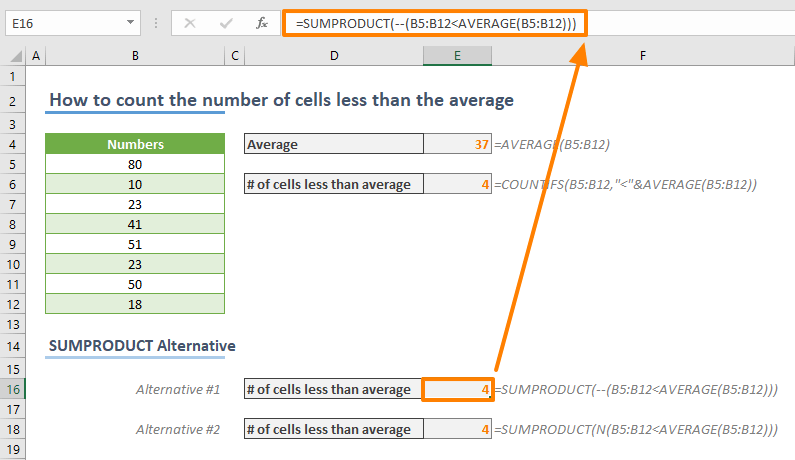In this article, we are going to show you how to count the number of cells that is less than the average of that data set in Excel.

## Formula

=COUNTIFS(<numbers range>,”<“&AVERAGE(<numbers range>))

<numbers range>: The reference from the range of numbers

## How it works

The formula relies on using the COUNTIFS and AVERAGE formulas. The COUNTIFS function can count cells that meet the given criteria. We can use a less than operator “<” (or any other operator) with the AVERAGE function as the criterion. Thus, there are two steps for counting the number of cells less than the average:

1. Calculate the average of numbers (AVERAGE)
2. Count the numbers less than the average (COUNTIFS)

Due to the need for a single criteria, alternatively, you can use the COUNTIF function instead of COUNTIFS.

A minor point to consider here is that you need to merge the less than operator with the AVERAGE formula as a string value “<”. Use ampersand “&” to merge.

Here is the formula for the range B5:B12:

=COUNTIFS(B5:B12,"<"&AVERAGE(B5:B12))## SUMPRODUCT Alternative

Now, let's look at an alternative approach to count the number of cells less than the average. For this method, we are going to be using SUMPRODUCT. The SUMPRODUCT function can evaluate arrays without dealing with array functions. With this, you can use the logical statement directly in the SUMPRODUCT function.

=SUMPRODUCT(--(B5:B12<AVERAGE(B5:B12)))

=SUMPRODUCT(N(B5:B12<AVERAGE(B5:B12)))

The B5:B12<AVERAGE(B5:B12) returns an array of Boolean values which indicate whether a value is less than the average. To convert the logical values into numbers (1 for TRUE, 0 for FALSE) you can use the unary operator “--" or N function.

Finally, the SUMPRODUCT function sums the 1 and 0 values to find the count the number of cells less than the average.Although the SUMPRODUCT alternative may not look easier to follow, but this approach has other advantages and also can help you better understand its capabilities.